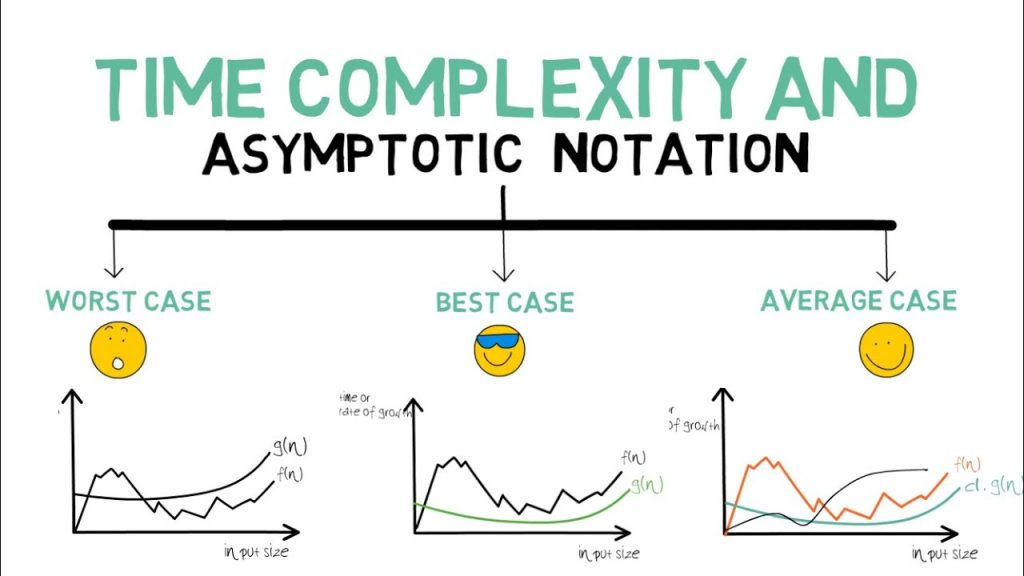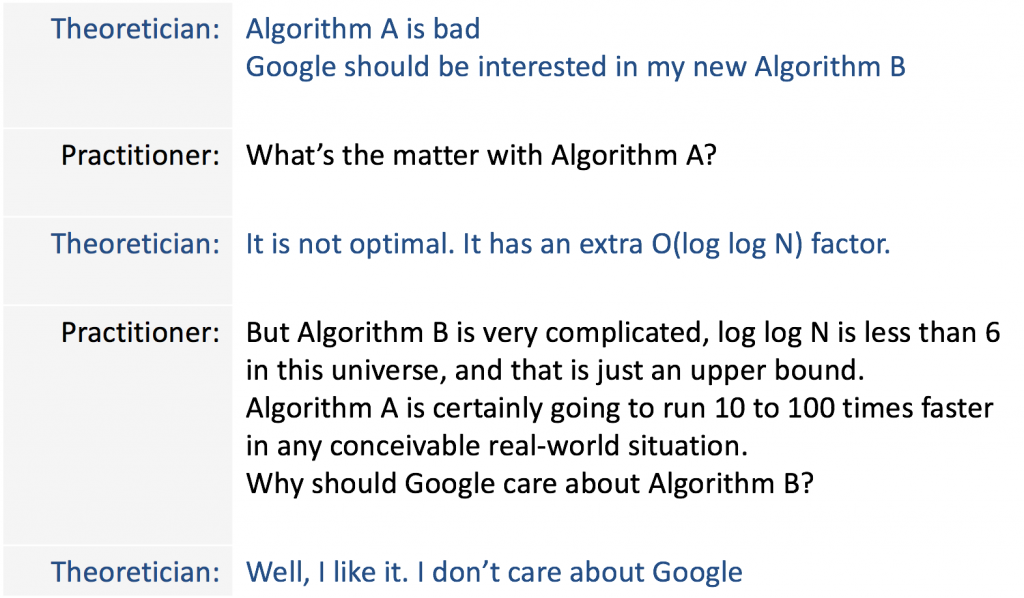# Motivation

What makes computers useful for us is primarily the ability to solve problems. The procedure in which computers solve a problem is an algorithm.  In the recent context of an increasing number of algorithms available for solving data-related problems, there is increasing demand for a higher level of understanding of algorithm’s performance for data scientists to choose the right algorithms for their problems.

Having a general perception of the efficiency of an algorithm would help to shape the thought process for creating or choosing better algorithms. With this intention in mind, I would like to create a series of posts to discuss what makes a good algorithm in practice, or for short, efficient algorithm. And this article is the first step of the journey.

# Define Efficiency

An algorithm is considered efficient if its resource consumption, also known as computational cost, is at or below some acceptable level. Roughly speaking, ‘acceptable’ means: it will run in a reasonable amount of time or space on an available computer, typically as a function of the size of the input.

There are many ways in which the resources used by an algorithm can be measured: the two most common measures are speed and memory usage. In the next 2 sections, we will be looking at the two different perspectives for measuring the efficiency of an algorithm from theoreticians and practitioners.

# Theoreticians perspective

Theoreticians are interested in measuring the efficiency of an algorithm without actually have to run it in several machines and input size. The key idea is that they are not going to consider the runtimes of the algorithm on any particular input. Rather, they look at what is known as asymptotic runtimes. Or in other words, they look at how the runtime scale with input size (n) as n gets larger. Does the output scale proportional to n, or proportional to n squared, or maybe exponential in n? These rates of growth are so different that as long as n is sufficiently large, constant multiples that come from other measures like temporary disk usage, long-term disk usage would be relatively small and neglected.Fig1:An illustration for time complexity using asymptotic notation for different functions

# Practitioner perspective

While certainly useful, the asymptotic runtime of an algorithm doesn’t tell the whole story. Some algorithms have good asymptotic runtime, but constants that are so huge that they effectively can’t be used. Ever. Lipton calls them Galactic Algorithms. A galactic algorithm is an algorithm that is wonderful in its asymptotic behavior but is never used to compute anything.Fig2: A fun exchange between a theoretician and practitioner

In practice, other factors that can affect the efficiency of an algorithm, such as requirements for accuracy and/or reliability. As detailed below, how an algorithm is implemented can also have a significant effect on actual efficiency, though many aspects of this relate to optimization issues.

Implementation issues can also effect efficiencies, such as the choice of programming language, or how the algorithm is coded, or the choice of a compiler for a particular language, or the compilation options used, or even the operating system being used. In many cases, a language implemented by an interpreter may be much slower than a language implemented by a compiler.